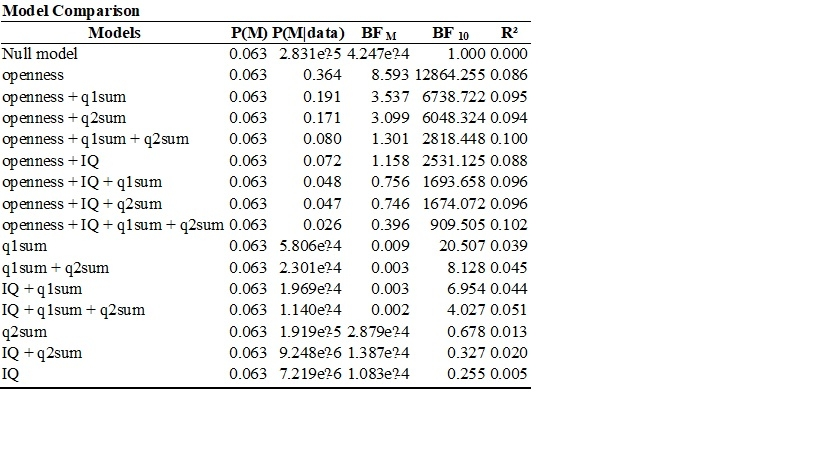#### Howdy, Stranger!

It looks like you're new here. If you want to get involved, click one of these buttons!

Supported by

# Interpreting BF10 and BFM in Bayesian linear regression

Hi,

I'm new to Bayesian statistics and JASP, and have a question about how to interpret the output from a multiple linear regression. Looking at the BF10, the model with openness as the only predictor is the strongest.

What does the BFM represent? There are three models with BFM > 3, so I am wondering whether I need to interpret this, and if so, how to do it. When I add the other predictors to the null model, the model with openness has a BF10 = 225, but the models with q1sum and q2sum each have BF10s < 1.

Thanks,

Sarah• BF_m quantifies the change from prior odds to posterior odds.
Here I'd select "compare to best model" and then BF_01 for display, and you'll see how many times better the "openness" only model predicts the data compared to other models.
Cheers,
E.J.

• Hello JASPers,

I am trying to understand how to compute the values in the Bayesian anova table below. I understand that P(M) means that the prior probabilities of the five models are equal and are obtained by 1/5. I also understand that P(M|data) refers to the posterior probability of each model after seeing the data. I also understand that BF M compares each model to the average P(M|data) of the other models. For example, the BF M of the DENSITY + SEASON Model can be obtained by     0.729/ (1- 0.729/4) = 0.729/ (0.271/4) = 0.729/0.06775 = 10.76. What I am trying to understand is how to obtain the values in BF 01. I selected BF 01 and Compare to best model and the Bayesian ANOVA table is shown below. Thanks in advance.

## Cheers,

narcilili

• hi again,

I think I got it regarding my question earlier today. I used the P(M|data) of the best model which is density + season as the numerator and the P(M|data) of the other models as the denominator. so, to compute BF01 for density + season + density*season I divided 0.729 by 0.251 yielding 2.9. but, dividing 0.729 by .001 yielded 729 instead of 576.852 when comparing the best model to the null model. maybe it is an approximation error?

cheers,

narcilili

• Dear Narcilili,

Yes, that's an approximation error. Note that your method works because the prior model probabilities are uniform. In general, the BF column compares the model in the top row ("0") to each of the models in the rows below ("1"). You selected "best model on top", so on the first row it is compared to itself, which yields BF=1 regardless of the data. The second row shows the BF in favor of the top-row model over the model in the second row (as BF_01, because that's what you selected). So the data are 2.9 times more likely under the two-main effect model than under the model that also includes the interaction.

Cheers,

E.J.

• Dear E.J.,

Thank you for your clear explanation. I would like to ask if it is possible to use Bayesian JASP for a randomized complete block design for two way ANOVA? Or an RCBD split-plot two way ANOVA? I would like to convince some agriculturists to use Baye's Factors. Thanks.

Cheers,

Narcilili

• Dear Narcilili,

Hmm I have not given this much thought. Perhaps the authors of the BayesFactor package have done this, and I will attend them to this question.

Cheers,

E.J.